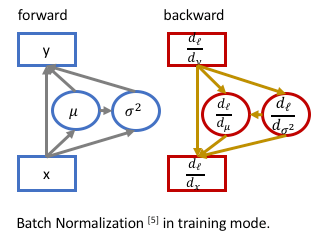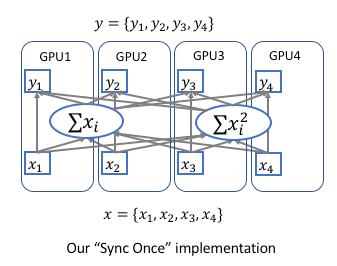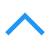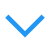# 深度学习网络层之 Batch Normalization

## Batch Normalization

S. Ioffe 和 C. Szegedy 在2015年《Batch Normalization: Accelerating Deep Network Training by Reducing Internal Covariate Shift》论文中提出此方法来减缓网络参数初始化的难处.

### Batch Norm原理

1）对特征的每个维度进行标准化，忽略白化中的去除相关性；
2）在每个mini-batch中计算均值和方差来替代整体训练集的计算。

batch normalization中即使不对每层的输入进行去相关，也能加速收敛。通俗的理解是在网络的每一层的输入都先做标准化预处理(公式中k为通道channel数),再向整体数据的均值方差方向转换.

$\text{unit gaussian activations} \\ \hat x^{(k)}=\frac{x^{(k)}-E[x^{(k)}]}{\sqrt{\operatorname{Var[x^{(k)}]}}}$

Batch Normalization转换式子:

\text{Batch Normalizing Transform} \\ \begin{align} \mu &= \frac{1}{m}\sum_{i=1}^m x_i &\text{//mini-batch mean} \\ \sigma^2 &= \frac{1}{m} \sum_{i=1}^m (x_i - \mu)^2 &\text{//mini-batch variance} \\ \hat{x_i} &= \frac{x_i - u}{\sqrt{\sigma^2 + \epsilon}} &\text{//normalize} \\ y_i &= \gamma \hat{x_i} + \beta &\text{//scale and shift} \end{align}

Batch Norm特征转换scale### 反向传播BN的前向与反向传播示意图:\begin{align} \bar\mu &=\mathbb E[\mu_\mathcal B] \\ \bar\sigma^2 &={m\over m-1}\mathbb E[\sigma^2_\mathcal B] \end{align}

\begin{align} y&=γ({x-\bar\mu\over\sqrt{\bar\sigma^2+ϵ}})+ β \\ &={γ\over\sqrt{\bar\sigma^2+ϵ}}\cdot x+(β-{γ\bar\mu\over\sqrt{\bar\sigma^2+ϵ}}) \end{align}

### Batch Norm优点

• 减轻过拟合
• 改善梯度传播（权重不会过高或过低）
• 容许较高的学习率，能够提高训练速度。
• 减轻对初始化权重的强依赖，使得数据分布在激活函数的非饱和区域，一定程度上解决梯度消失问题。
• 作为一种正则化的方式，在某种程度上减少对dropout的使用。

### Batch Norm 应用

Batch Norm在卷积层的应用

1. 重新完全训练.如果想将BN添加到卷基层，通常要重新训练整个模型，大概花费一周时间。
2. finetune.只将BN添加到最后的几层全连接层，这样可以在训练好的VGG16模型上进行微调。采用ImageNet的全部或部分数据按batch计算均值和方差作为BN的初始$\beta,\gamma$参数。

### 与 Dropout 合作

Batch Norm的提出使得dropout的使用减少,但是Batch Norm不能完全取代dropout,保留较小的dropout率,如0.2可能效果更佳。

## Batch Norm 实现

caffe的BatchNorm层参数设置示例:

layer {
name: "conv1/bn"
type: "BatchNorm"
bottom: "conv1"
top: "conv1"
param { lr_mult: 0 decay_mult: 0 } # mean
param { lr_mult: 0 decay_mult: 0 } # var
param { lr_mult: 0 decay_mult: 0 } # scale
batch_norm_param { use_global_stats: true } # 训练时设置为 false
}


caffe框架中 BN 层全局均值和方差的实现

\begin{align} \mu_{new} &= (\lambda \mu_{old} + \mu_B) / s \\ \sigma_{new}^2 &= \begin{cases} (\lambda \sigma_{old}^2 + \frac{m - 1}{m} \sigma_B^2)/s & m > 1 \\ (\lambda \sigma_{old}^2 + \sigma_B^2)/s & m = 1 \end{cases} \\ s &= \lambda s+1 \end{align}

caffe中的batch_norm_layer仅含均值方差,不包括gamma/beta,需要后边紧跟scale_layer,且使用bias对应beta.scale层用于自动学习缩放参数,同时包括了bias_layer能够学习bias.


// scale初始化代码: 用三个blob记录BatchNorm层的三个数据
void BatchNormLayer<Dtype>::LayerSetUp(...) {
vector<int> sz;
sz.push_back(channels_);
this->blobs_.reset(new Blob<Dtype>(sz)); // mean
this->blobs_.reset(new Blob<Dtype>(sz)); // variance
// 在caffe实现中计算均值方差采用了滑动衰减方式, 用了scale_factor代替num_bn_samples(scale_factor初始为1, 以s=λs + 1递增).
sz = 1;
this->blobs_.reset(new Blob<Dtype>(sz)); // normalization factor (for moving average)
}

if (use_global_stats_) {
// use the stored mean/variance estimates.
const Dtype scale_factor = this->blobs_->cpu_data() == 0 ?
0 : 1 / this->blobs_->cpu_data();
caffe_cpu_scale(variance_.count(), scale_factor,
this->blobs_->cpu_data(), mean_.mutable_cpu_data());
caffe_cpu_scale(variance_.count(), scale_factor,
this->blobs_->cpu_data(), variance_.mutable_cpu_data());
} else {
// compute mean
caffe_cpu_gemv<Dtype>(CblasNoTrans, channels_ * num, spatial_dim,
1. / (num * spatial_dim), bottom_data,
spatial_sum_multiplier_.cpu_data(), 0.,
num_by_chans_.mutable_cpu_data());
caffe_cpu_gemv<Dtype>(CblasTrans, num, channels_, 1.,
num_by_chans_.cpu_data(), batch_sum_multiplier_.cpu_data(), 0.,
mean_.mutable_cpu_data());
}

// subtract mean
caffe_cpu_gemm<Dtype>(CblasNoTrans, CblasNoTrans, num, channels_, 1, 1,
batch_sum_multiplier_.cpu_data(), mean_.cpu_data(), 0.,
num_by_chans_.mutable_cpu_data());
caffe_cpu_gemm<Dtype>(CblasNoTrans, CblasNoTrans, channels_ * num,
spatial_dim, 1, -1, num_by_chans_.cpu_data(),
spatial_sum_multiplier_.cpu_data(), 1., top_data);

if (!use_global_stats_) {
// compute variance using var(X) = E((X-EX)^2)
caffe_powx(top->count(), top_data, Dtype(2),
temp_.mutable_cpu_data());  // (X-EX)^2
caffe_cpu_gemv<Dtype>(CblasNoTrans, channels_ * num, spatial_dim,
1. / (num * spatial_dim), temp_.cpu_data(),
spatial_sum_multiplier_.cpu_data(), 0.,
num_by_chans_.mutable_cpu_data());
caffe_cpu_gemv<Dtype>(CblasTrans, num, channels_, 1.,
num_by_chans_.cpu_data(), batch_sum_multiplier_.cpu_data(), 0.,
variance_.mutable_cpu_data());  // E((X_EX)^2)

// compute and save moving average
this->blobs_->mutable_cpu_data() *= moving_average_fraction_;
this->blobs_->mutable_cpu_data() += 1;
caffe_cpu_axpby(mean_.count(), Dtype(1), mean_.cpu_data(),
moving_average_fraction_, this->blobs_->mutable_cpu_data());
int m = bottom->count()/channels_;
Dtype bias_correction_factor = m > 1 ? Dtype(m)/(m-1) : 1;
caffe_cpu_axpby(variance_.count(), bias_correction_factor,
variance_.cpu_data(), moving_average_fraction_,
this->blobs_->mutable_cpu_data());
}

// normalize variance
caffe_powx(variance_.count(), variance_.cpu_data(), Dtype(0.5),
variance_.mutable_cpu_data());

// replicate variance to input size
caffe_cpu_gemm<Dtype>(CblasNoTrans, CblasNoTrans, num, channels_, 1, 1,
batch_sum_multiplier_.cpu_data(), variance_.cpu_data(), 0.,
num_by_chans_.mutable_cpu_data());
caffe_cpu_gemm<Dtype>(CblasNoTrans, CblasNoTrans, channels_ * num,
spatial_dim, 1, 1., num_by_chans_.cpu_data(),
spatial_sum_multiplier_.cpu_data(), 0., temp_.mutable_cpu_data());
caffe_div(temp_.count(), top_data, temp_.cpu_data(), top_data);

caffe_copy(x_norm_.count(), top_data,
x_norm_.mutable_cpu_data());


BN有合并式和分离式，各有优劣。

### BatchNorm层合并(Conv+BN+Scale+ReLU => Conv+ReLU)

bn layer: bn_mean, bn_variance, num_bn_samples 注意在caffe实现中计算均值方差采用了滑动衰减方式,用了scale_factor代替num_bn_samples(scale_factor初始为1,以s=λs+1递增).
scale layer: scale_weight, scale_bias 代表gamma,beta
BN层的batch的均值mu=bn_mean/num_bn_samples,方差var=bn_variance / num_bn_samples.
scale层设置新的仿射变换参数:

new_gamma = gamma / (np.power(var, 0.5) + 1e-5)
new_beta = beta - gamma * mu / (np.power(var, 0.5) + 1e-5)


Conv+BN+Scale=>Conv
conv layer: conv_weight, conv_bias

alpha = scale_weight / sqrt(bn_variance / num_bn_samples + eps)
conv_bias = conv_bias * alpha + (scale_bias - (bn_mean / num_bn_samples) * alpha)
for i in range(len(alpha)): conv_weight[i] = conv_weight[i] * alpha[i]


## Batch Norm 多卡同步

BatchNorm的实现都是只考虑了single gpu。也就是说BN使用的均值和标准差是单个gpu算的，相当于缩小了mini－batch size。至于为什么这样实现，1）因为没有sync的需求，因为对于大多数vision问题，单gpu上的mini-batch已经够大了，完全不会影响结果。2）影响训练速度，BN layer通常是在网络结构里面广泛使用的，这样每次都同步一下GPUs，十分影响训练速度。\begin{align} \mu &= \frac{1}{m}\sum_{i=1}^m x_i \\ \sigma^2 &= \frac{1}{m} \sum_{i=1}^m (x_i - \mu)^2 = \frac{1}{m} \sum_{i=1}^m (x_i^2+\mu^2-2x_i\mu) = \frac{1}{m} \sum_{i=1}^m x_i^2 - \mu^2 \\ &= \frac{1}{m} \sum_{i=1}^m x_i^2 - (\frac{1}{m}\sum_{i=1}^m x_i)^2 \end{align}

posted @ 2017-09-22 19:55  康行天下  阅读(15367)  评论(11编辑  收藏  举报